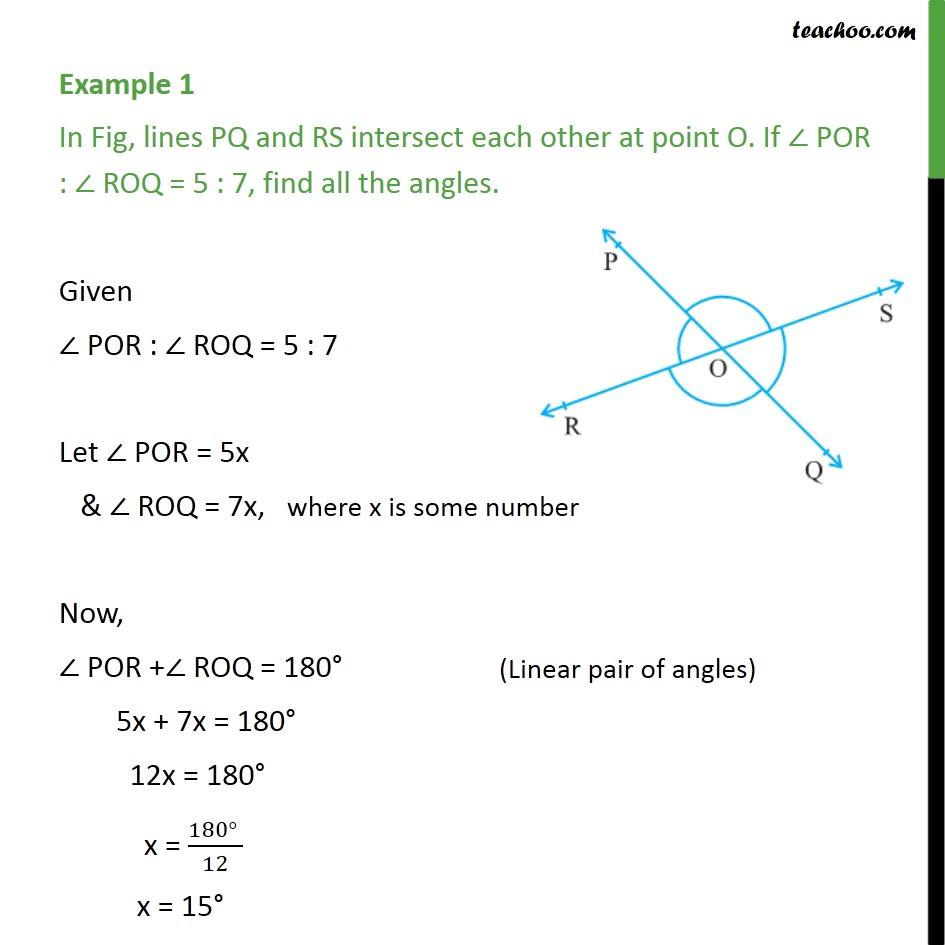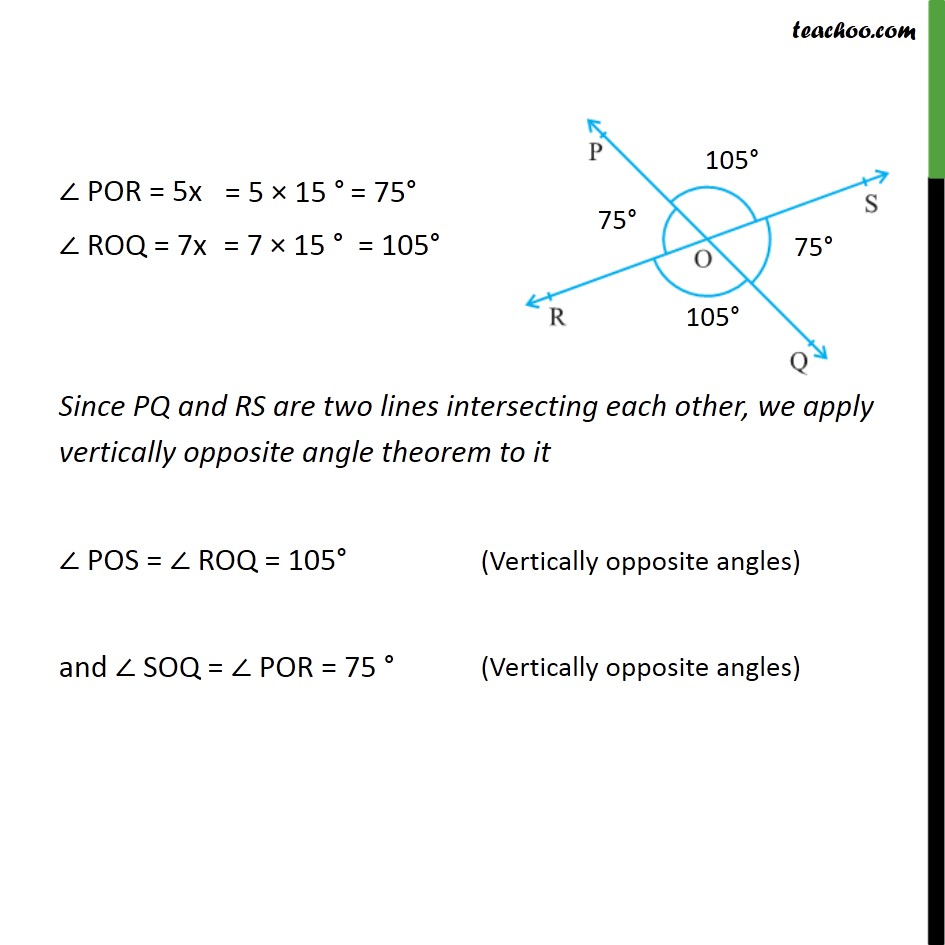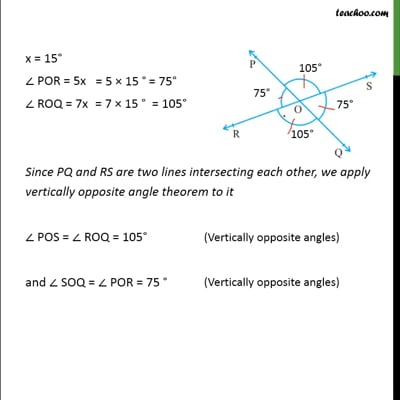Examples

Chapter 6 Class 9 Lines and Angles
Serial order wiseThis video is only available for Teachoo black users

Maths Crash Course - Live lectures + all videos + Real time Doubt solving!

### Transcript

Example 1 In Fig, lines PQ and RS intersect each other at point O. If ∠ POR : ∠ ROQ = 5 : 7, find all the angles. Given ∠ POR : ∠ ROQ = 5 : 7 Let ∠ POR = 5x & ∠ ROQ = 7x, where x is some number Now, ∠ POR +∠ ROQ = 180° 5x + 7x = 180° 12x = 180° x = (180° )/12 x = 15° ∠ POR = 5x ∠ ROQ = 7x Since PQ and RS are two lines intersecting each other, we apply vertically opposite angle theorem to it ∠ POS = ∠ ROQ = 105° and ∠ SOQ = ∠ POR = 75 °